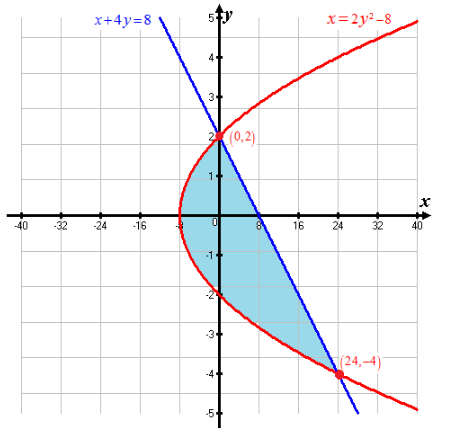# To sketch the region bounded by the curves### Single Variable Calculus: Concepts...

4th Edition
James Stewart
Publisher: Cengage Learning
ISBN: 9781337687805### Single Variable Calculus: Concepts...

4th Edition
James Stewart
Publisher: Cengage Learning
ISBN: 9781337687805

#### Solutions

Chapter B, Problem 53E
To determine

## To calculate: To sketch the region bounded by the curves

Expert Solution

The region bounded by the curves is sketched

### Explanation of Solution

Given information: Equation of curves are x+4y=8 and x=2y28

Calculation:

Equation of curves are given as follows:

x+4y=8 --------(1)

x=2y28 -------(2)

Substituting the value of x=2y28 in equation (1)

2y28+4y=82y2+4y16=0y2+2y8=0y2+4y2y8=0y(y+4)2(y+8)=0(y+4)(y2)=0y=4,2

Now, substituting the values of y=4 in equation (1)

x+4y=8x+4(4)=8x=8+16x=24

Thus, one point of intersection is (24,4)

Again, substituting the values of y=2 in equation (1)

x+4y=8x+4(2)=8x=88x=0

Thus, one point of intersection is (0,2)

Hence the area between the curves is displayed as follows:Conclusion:

Hence, region bounded by the curves is sketched

### Have a homework question?

Subscribe to bartleby learn! Ask subject matter experts 30 homework questions each month. Plus, you’ll have access to millions of step-by-step textbook answers!